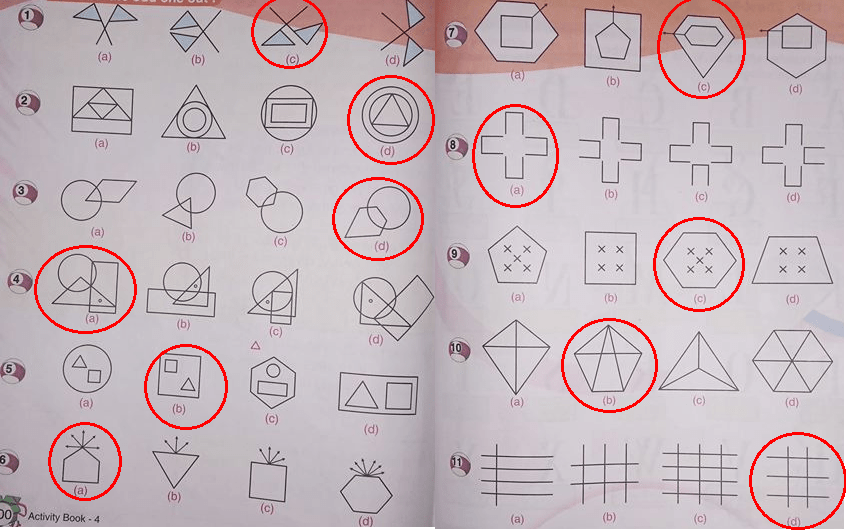# RS Aggarwal Vikash Aggarwal Class 4 Activity Book Composite Mathematics Activity Sheet 87 Solution

## Activity Sheet – 87

### Choose the odd one out:(1) (c): This is the only figure in which the two flags point in the same direction.

(2) (d): In all other figures, the two inner figures are identical.

(3) (d) : In all other figures, only one vertex of the other figure is inside the circle.

(4) (a) : In all other figures, the dot lies inside the circle and triangle but outside the rectangle.

(5) (b) : This is the only figure in which one inner figure is identical to the outer figures.

(6) (a): In all other figures, the arrows are attached to a side of the main figure.

(7) (c) : This is the only figure in which the arrow passes through the vertices of both the inner and outer figures.

(8) (a) : In all other figures, one cup faces outward.

(9) (c) : In all other figures, the number of crosses is equal to the numbert of sides in the figure.

(10) (b) : Each other figure is divided into as many parts as is the number of sides in the figure.

(11) (d) : In all other figures, there is an even number of horizontal lines and an odd number of vertical lines.

Updated: January 2, 2019 — 2:48 pm